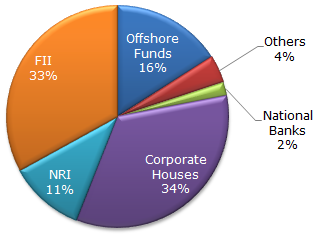# Data Interpretation - Pie Charts - Discussion

The following pie chart shows the amount of subscriptions generated for India Bonds from different categories of investors.

Subscriptions Generated for India Bonds5.

If the total investment flows from FII's were to be doubled in the next year and the investment flows from all other sources had remained constant at their existing levels for this year, then what would be the proportion of FII investment in the total investment into India Bonds next year (in US \$ millions) ?

 [A]. 40 % [B]. 50 % [C]. 60 % [D]. 70 %

Explanation:

FII's currently account for 33 out of 100.

If their value is doubled and all other investments are kept constant then their new value would be 66 out of 133 = approximately equal to 50%

 V P Elakkiya said: (Dec 10, 2014) How it's equal to 50%?

 Nandaraj said: (May 6, 2015) 66 out of 133 = 66/133*100%. = 1/2*100%. = 50%. Hope you understand.

 Kitty said: (Sep 14, 2015) How it is 133?

 Preti said: (Nov 21, 2015) As only the FII is doubled and not all the other sources. So current FII is 33 out of 100. So doubling it, will become 100+33 = 133.

 Thete Sanket said: (Mar 30, 2019) Thanks @Nandraj.

 Sohel said: (Dec 25, 2019) How it is 66?